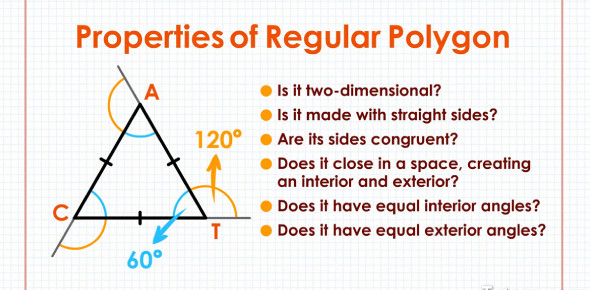# Section 7.2 - Angle Properties Of Polygons

Approved & Edited by ProProfs Editorial Team
At ProProfs Quizzes, our dedicated in-house team of experts takes pride in their work. With a sharp eye for detail, they meticulously review each quiz. This ensures that every quiz, taken by over 100 million users, meets our standards of accuracy, clarity, and engagement.
| Written by Seixeiroda
S
Seixeiroda
Community Contributor
Quizzes Created: 44 | Total Attempts: 21,177
Questions: 9 | Attempts: 709SettingsIncase you fancy yourself a math fanatic and are up to answering queries about angle properties of polygons try out this quiz. It is a simple set of questions therefore it will be easy to answer. All the best.

• 1.

### Which shows an exterior angle

• A.

W

• B.

X

• C.

Y

• D.

Z

D. Z
Explanation
The correct answer is z because an exterior angle is formed when a side of a polygon is extended. In this case, z is the only angle that is outside the polygon and formed by extending one of its sides.

Rate this question:

• 2.

### In triangle LMN, the measure of ÐM is 4° more than twice the measure of Ð L.  The measure of Ð N is 27° less than half the measure of Ð L.  What is the measure of Ð N?

• A.

2 degrees

• B.

13.6 degrees

• C.

29 degrees

• D.

56 degrees

A. 2 degrees
Explanation
The measure of angle N is 27 degrees less than half the measure of angle L. Since the measure of angle L is not given, we cannot determine the exact measure of angle N. Therefore, we cannot confirm that the given answer of 2 degrees is correct.

Rate this question:

• 3.

### What is the meansure of ÐACB is the diagram?

• A.

48 degrees

• B.

78 degrees

• C.

102 degrees

• D.

126 degrees

B. 78 degrees
Explanation
The measure of angle ACB in the diagram is 78 degrees.

Rate this question:

• 4.

### Brian has been making octagonal wooden puzzles.  He wants to make some hexagonal puzzles next.  By how much does he need to increase or decrease the interior angle measure on his template to make the hexagonal puzzles?

• A.

Increase by 15 degrees

• B.

Decrease by 15 degrees

• C.

Increase by 35 degrees

• D.

Decrease by 35 degrees

B. Decrease by 15 degrees
Explanation
To make hexagonal puzzles, Brian needs to decrease the interior angle measure on his template by 15 degrees. This is because an octagon has interior angles measuring 135 degrees, while a hexagon has interior angles measuring 120 degrees. Therefore, by decreasing the angle measure by 15 degrees, Brian will be able to create hexagonal puzzles.

Rate this question:

• 5.

### What is the sum of the exterior angles in a regular 13-gon?

• A.

152.3 degrees

• B.

360 degrees

• C.

1980 degrees

• D.

3960 degrees

B. 360 degrees
Explanation
The sum of the exterior angles in any polygon, including a regular 13-gon, is always 360 degrees. This is a property of polygons, where the sum of the exterior angles is equal to a full rotation of 360 degrees. Therefore, the correct answer is 360 degrees.

Rate this question:

• 6.

### Home plate on a baseball diamond has 3 right angles.  What is the sum of the measure of Ðx and Ðy

• A.

45 degrees

• B.

90 degrees

• C.

135 degrees

• D.

270 degrees

B. 90 degrees
Explanation
Home plate on a baseball diamond is a square shape, with each corner forming a right angle. Since it is mentioned that there are 3 right angles, it means that there is only one angle that is not a right angle. Therefore, the sum of the measure of Ðx and Ðy must be 90 degrees, as the only way to have 3 right angles in a square is if the remaining angle is also 90 degrees.

Rate this question:

• 7.

### What is the measure of angle x in this diagram?

• A.

60 degrees

• B.

80 degrees

• C.

120 degrees

• D.

240 degrees

A. 60 degrees
Explanation
The measure of angle x in the diagram is 60 degrees.

Rate this question:

• 8.

### Which equation expresses the relationship needed to solve for x?

• A.

7x = 322

• B.

X + 2x + (x + 29) + (3x + 9) = 380

• C.

7x + 38 = 180

• D.

7x = 398

A. 7x = 322
Explanation
The equation 7x = 322 expresses the relationship needed to solve for x. This equation states that 7 times x is equal to 322.

Rate this question:

• 9.

### What is the measure of an exterior angle of a regular polygon in which the sum of the interior angle measures is 5400 °

• A.

11.25 degrees

• B.

30 degrees

• C.

32 degrees

• D.

168.75 degreesBack to top